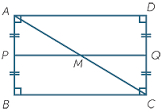# The length 4

The length of a rectangular room measures twice a number x increased by 5. The width measures a number x increased by 3. What is the perimeter of the room?

Result

p = (Correct answer is: 4x+16)### Step-by-step explanation:Did you find an error or inaccuracy? Feel free to write us. Thank you!

Tips for related online calculators
Do you want to convert length units?

#### Grade of the word problem:

We encourage you to watch this tutorial video on this math problem: